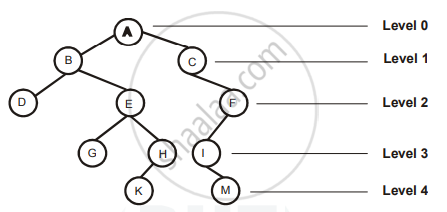# With Suitable Example Show the Relationship Between Total Number of Nodes and Depth of Binary Tree. - Computer Science 1

With suitable example show the relationship between Total Number of Nodes and Depth of Binary Tree.

#### Solution

Each node in a tree is assigned a level number. Generally, the level number of root R of the tree is zero and every other node is assigned to the level number which is one more than the level number of its parent. It is the distance from the root.Depth of a binary tree:-Depth of a binary tree is defined as the maximum level of any nodes in the tree.

The depth of the binary tree is equal to 1+ largest level number.

The maximum number of nodes of a symmetric binary tree with depth n is 2n -1.

E.g. The depth of above binary tree =1+largest level number =1+4=5

Maximum number of nodes=25-1=32-1=31.

(In above diagram all the nodes are not shown.)

Concept: Basic Data Structures (Stack, Queue, Dequeue)
Is there an error in this question or solution?